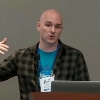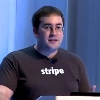InfoQ Homepage Presentations Category Theory for the Working Hacker

# Category Theory for the Working Hacker

## Key Takeaways

• There are deep connections between logic, lambda calculus, and category theory.
• In category theory we've got objects and arrows. An object in category theory is a type. Arrows are functions that have an argument of a given type and return a result of a given type.
• The three most important structures in programming (records, variant records, and functions) arise in category theory (product, sum, exponential) and their correspondence with the three most important construct in logic (and, or, implication).
• In category theory a function, that is an arrow, can also be an object. This corresponds to the most profound idea in programming: that programs are also data.
• All of this was built to explain deep connections between lambda calculus and ideas from logic. You should prefer using a programming language based on lambda calculus.

## Show notes

• 1:54 Formal logic and lambda calculus were both formalized in the 1930s, and after 50 years, in 1980, William A. Howard published a paper saying they are exactly the same thing.
• 2:26 The fact that Gerhart Gentzen and Alonzo Church both came up with the same idea but it took about 40 years before people recognize that they are the same idea, then it took another 10 years for Howard to get around to publishing it, is what makes us say that they had not invented something, but they had discovered something.
• 3:34 There are deep connections between logic, lambda calculus, and category theory, which is the focus on this presentation.
• 4:55 In category theory we've got objects and arrows. An object in category theory is a type. Arrows are functions that have an argument of a given type and return a result of a given type.
• 5:32 There are many instances of this kind of renaming: in set theory, objects are sets and arrows can be functions between the sets or relations between the sets; in domain theory, objects are domains and arrows are again functions between the domains; in abstract algebra, objects are groups and arrows are morphisms between groups.
• 6:50 Two simple notions that will be used in the remainder of the talk are those of identity function and function composition. The identity function is a function that simply returns its argument. Function composition allows to take a function `f` from `A` to `B` and another function `g` from `B` to `C` and create a third function `f;g` (also denoted by `g.f`) from `A` to `C`.
• 8:10 Function composition is governed by some laws. The first law says the identity function, `id`, followed by `f` is the same as `f`.
• 9:00 Another law states that if you compose three functions, it doesn't matter what order you compose them, i.e., composition is associative.
• 9:26 This talk will show how the three most important structures in programming (records, variant records, and functions) arise in category theory (product, sum, exponential) and their correspondence with the three most important construct in logic (and, or, implication).

Product

• 9:50 Given an object `A` and an object `B`, we form the product `A x B` (A times B). In set theory, this would be Cartesian product; in programming, tuple record with two fields, the first of type `A` and the second of type `B`.
• 10:45 Let's call `fst` a function which given an `A x B` pair returns the `A` component and `snd` a function which given an `A x B` pair returns the `B` component.
• 12:00 If `f` is a function from `C` to `A` and `g` is a function from `C` to `B`, I can build a pair from that - let's call this function .
• 12:30 is how you build a pair. `fst` and `snd` are how you take it apart.• 12:46 The diagram shown above, called a commutative diagram, tells us that if you build a pair using and you extract its `fst` component, that is the same as using `f` alone. Similarly, using and then `snd` to get the second component of the pair, is the same as using `g` alone. This is expressed in the following isomorphism:• 13:25 The above property only holds if `f` and `g` have no side effects. This is an argument against having side effect, as it gives you useful and simple laws.
• 14:12 The dotted line in the diagram as a special meaning, i.e., if a function makes this diagram commute, it is unique.
• 14:30 A commuting diagram means that if there are two different pathways from one place to another that involve applying different functions, they must be equivalent.
• 15:30 In short, what the diagram tells us is: you can take pairs apart using `fst` and `snd`; you can build pairs using ; those operations are inverse to each other and is the unique thing that makes this work.
• 15:51 Being unique important yet there are all sorts of things that you can work out from that are really useful like you can prove things (example follows)
• 21:40 The product corresponds to the `and` logical operator.

Sum

• 23:00 Another major data structure is the sum, which is sometimes called a disjoint union, or record variants in Pascal, either in Haskell, etc.
• 24:00 To build a disjoint union, given an `A` we can inject it into the type `A+B`. This could be represented in a particular case as something with a numeric tag field followed by a value, which could be of a different type depending on the tag.
• 24:49 We can build one of these sum values using two functions: `inl` (in-left) and `inr` (in-right). We can take it apart doing case analysis. If `f` is a function from `A` to `C` and `g` is a function from `B` to `C`, we can get a `C` the sum `A+B` using `[f,g]`, which looks at the concrete type in the sum and, if it is an `A`, applies `f` to it; if it's a `B`, it applies `g` to it.• 25:18 The commutative diagram for a sum tells us that if you have `inl` followed by `[f,g]`, that is the same as `f`; if you have `inr` followed by `[f,g]` that is the same as `g`. Again the dotted line means that `[f,g]` is universal, meaning that if you have some `h` that makes this diagram commute you know that that's the same as `[f,g]`.
• 27:50 Using the commuting diagram for `A+B`, it is possible to prove the following isomorphism:which means that having an arrow from an `A+B` to `C` and precomposing it using either `inl` or `inr` is the same as having an arrow from an `A` to `C` and having an arrow from `B` to `C`.

• 31:00 We can define an additional contract for the sum, called distributivity:• 31:35 Sum types correspond to disjunction in logic, that is the `or` operator.

Exponential

• 32:35 The exponential leads us to currying, i.e., to the concept of higher-order functions. In this case the commutative diagram shows the following isomorphism:•• 34:40 The commutative diagram for exponential, show how you can go from a product of two objects to a product that includes as its first term a functionWhat this means is that in category theory a function, that is an arrow, can also be an object. This corresponds to the most profound idea in programming: that programs are also data. Not every data is a program, but every program can be converted into data.
• 37:30 Exponential corresponds to implication in logic.
• 37:40 Product, sum, and exponential represent the three most important concepts in programming: the record, the variant record, and the function.
• 38:00 The commutative diagrams for products and sums have an interesting relation: they are just the same diagram with reversed arrows. They are dual.
• 41:24 All of this was built to explain the semantics of lambda calculus and to explain deep connections between programming languages based on lambda calculus and logical ideas for natural deduction.

## Resources

• Howard, William A. (September 1980) [original paper manuscript from 1969], "The formulae-as-types notion of construction", in Seldin, Jonathan P.; Hindley, J. Roger, To H.B. Curry: Essays on Combinatory Logic, Lambda Calculus and Formalism, Boston, MA: Academic Press, pp. 479-490, ISBN 978-0-12-349050-6.

## See more presentations with show notesSafe Systems Programming in C# and .NETScaling Uber to 1,000 ServicesRust: Systems Programming for Everyone by Felix KlockThe Architecture that Helps Stripe Move Faster

Jul 28, 2016

## Hello stranger!

You need to Register an InfoQ account or or login to post comments. But there's so much more behind being registered.

Get the most out of the InfoQ experience.

Allowed html: a,b,br,blockquote,i,li,pre,u,ul,p

## Community comments

• ##### I agree - it was cool

by Richard Richter /

• ##### I agree - it was cool

Your message is awaiting moderation. Thank you for participating in the discussion.

This is probably the second presentation with categories I've seen and I believe it was better. Maybe because some of it sank ink after the first one some time ago - but I think the explanations here were really better. However, the slides don't follow the talk very well so it seems, so it still is hard to follow. I'm sure it's not my last category theory presentation I'd see, because somehow it fascinates me. :-)

Allowed html: a,b,br,blockquote,i,li,pre,u,ul,p

Allowed html: a,b,br,blockquote,i,li,pre,u,ul,p

Is your profile up-to-date? Please take a moment to review and update.

Note: If updating/changing your email, a validation request will be sent

Company name:
Company role:
Company size:
Country/Zone:
State/Province/Region:
You will be sent an email to validate the new email address. This pop-up will close itself in a few moments.